#### How to Calculate Skewness in Excel

In this Excel tutorial lesson, you will learn what skewness is and how to calculate skewness in an Excel application.

## Definition

Skewness is the statistic by which you define asymmetry in the distribution of the analyzed variable. With skewness, you’ll see how the results of your variable shape around the average. If their distribution is similar to the normal distribution (skewness equals to 0) or whether you can deal with the distribution positively skewed (skewness > 0) or negatively skewed (skewness <0).

To find the skewness in Excel, please prepare some data tables.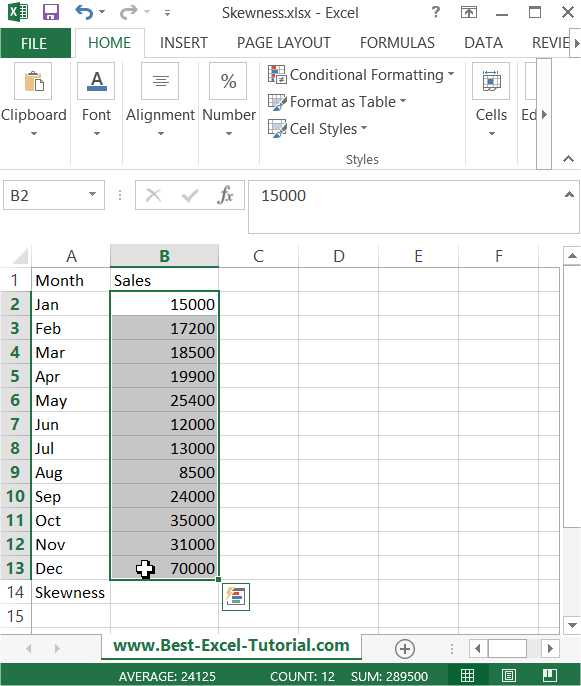## Calculate Skewness using SKEW function

Excel provides a SKEW function. Syntax is:

=SKEW(number1, [number2], …)

In this example, the formula is: =SKEW(B2:B13)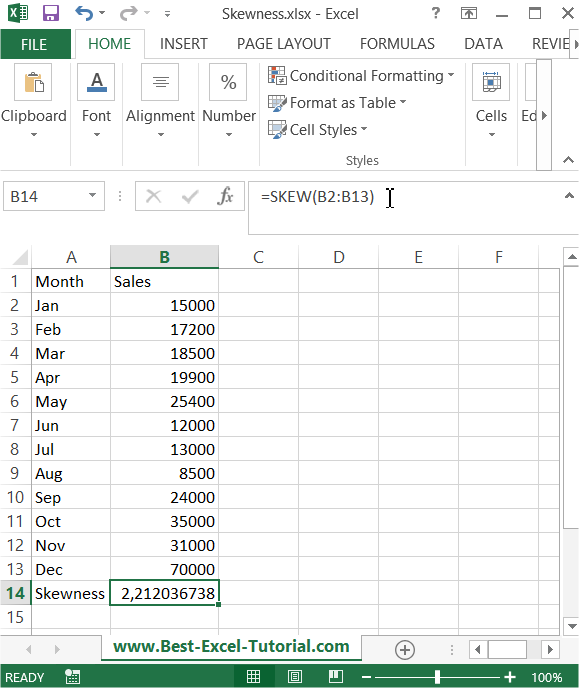Skewness here is strongly above 0 (skewness > 0), which means that it is a right-sided asymmetry. The conclusion is that for many months, sales were significantly lower than the average sales.

## Calculate Skewness using Data Analysis ToolPak Add-In

First, you need to make sure your Data Analysis Toolpak Add-In is installed. Here is the lesson on how to install Data Analysis Toolpak Add-In.

Go to Ribbon to the Data tab. Click the Data Analysis button.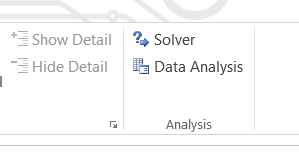A new window appears. Choose Descriptive Statistics and click OK.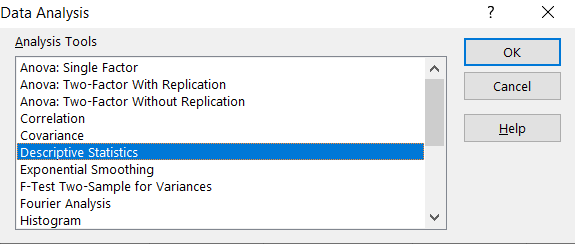Enter the proper parameters:

• Input Range – range of your data (\$B\$1:\$B\$13 in my example).
• Labels in first row – there are labels in my data table.
• Output Range – choose where you want to get your skewness calculated (I chose the same Sheet \$E\$1:\$F\$15).
• Summary statistics – make sure it is checked.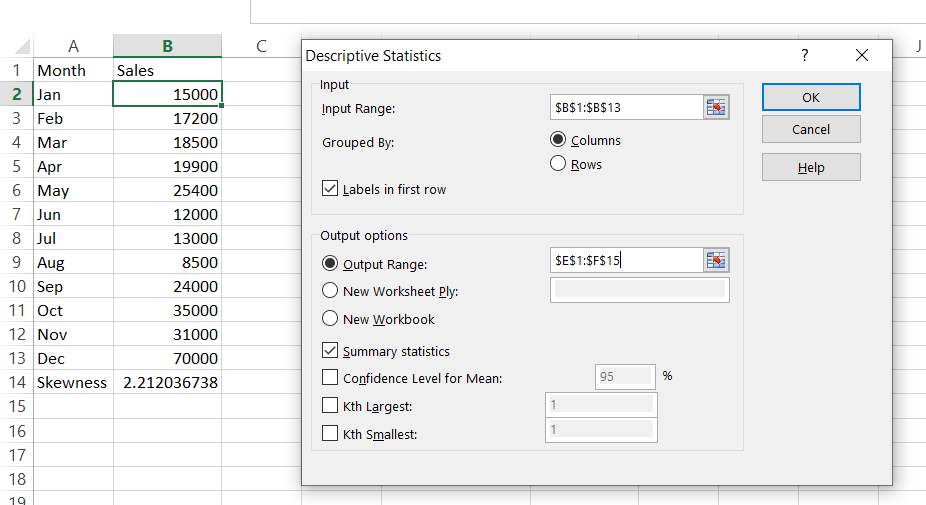And this is how you see my statistics summary. Skewness is calculated in row 10. The result is the same as in Example 1 where we checked how to calculate Skewness using the SKEW Excel function.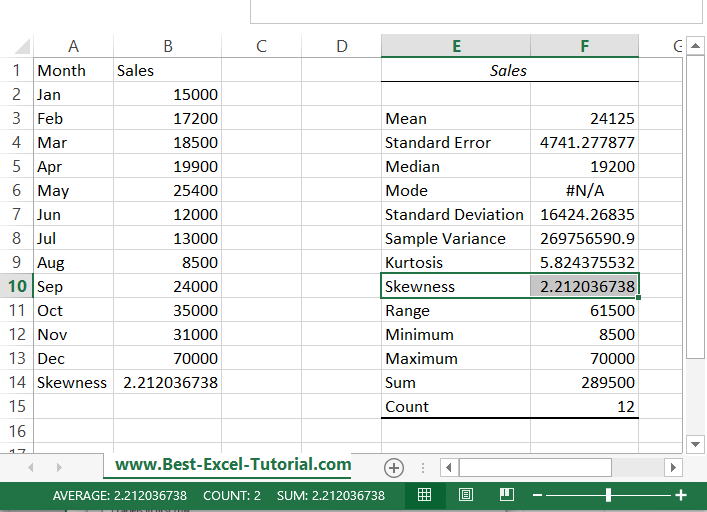## Using the STDEV.P function and the AVG function

Another way to calculate skewness in Excel is to use the STDEV.P function and the AVG function. To do this, you need to calculate the standard deviation and the mean of the data first, and then use the following formula to calculate skewness:

Skewness = 3 * (AVG – Mean) / STDEV.P

Where AVG represents the average of the data, Mean represents the mean of the data, and STDEV.P represents the standard deviation of the data.

## The third moment of the data

Another way to calculate skewness in Excel is to use the formula for skewness that is based on the third moment of the data. The third moment measures the amount of skewness in the distribution. The formula for skewness is as follows:

Skewness = (N / (N – 1) * (N – 2)) * SUM((X – Mean)^3) / (STDEV.P^3)

Where:

• N is the number of data points
• X is the value of each data point
• Mean is the average of the data
• STDEV.P is the standard deviation of the data calculated by the STDEV.P function
• SUM is the SUM function in Excel, which calculates the sum of a range of values

To use this formula in Excel, you first need to calculate the mean, standard deviation, and the sum of the cubed deviations from the mean. Once you have these values, you can use the formula to calculate the skewness.

This method provides a more detailed and accurate representation of skewness in your data, as it takes into account the third moment of the distribution. However, it requires more calculation steps than the other methods and may be more complex for those who are new to statistical analysis in Excel.

## Formula based on the standardized score or z-score of each data point

The last way I know to calculate skewness in Excel is to use the formula based on the standardized score or z-score of each data point. The formula for skewness using z-scores is as follows:

Skewness = SUM(Z^3) / N

Where:

• Z is the z-score of each data point, which is calculated as (X – Mean) / STDEV.P
• N is the number of data points
• X is the value of each data point
• Mean is the average of the data
• STDEV.P is the standard deviation of the data calculated by the STDEV.P function
• SUM is the SUM function in Excel, which calculates the sum of a range of values

To use this formula in Excel, you first need to calculate the mean, standard deviation, and the z-scores for each data point. Once you have these values, you can use the formula to calculate the skewness.

This method provides a simpler and more intuitive way to calculate skewness, as it standardizes the data to help eliminate the effects of different scale and units of measurement. However, it may not provide as accurate a representation of skewness compared to the formula based on the third moment of the data, as it only takes into account the first and second moments of the distribution.